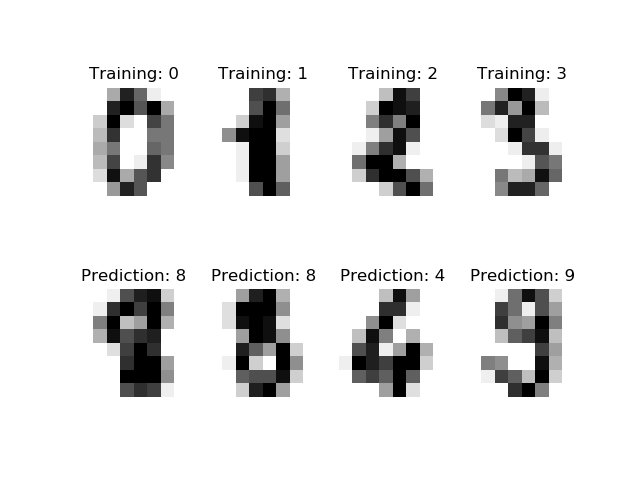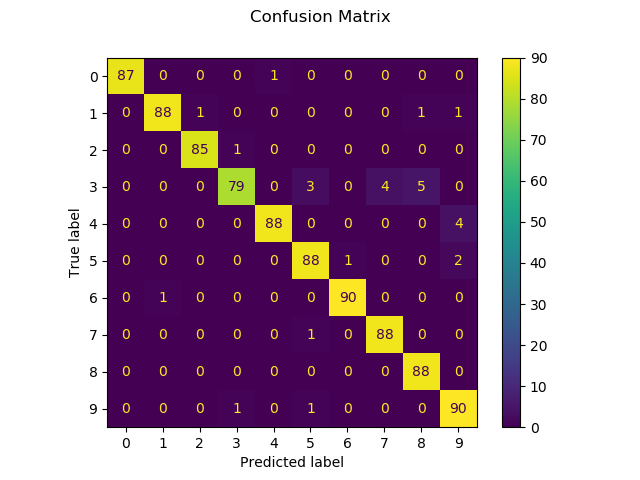# Recognizing hand-written digits¶

An example showing how the scikit-learn can be used to recognize images of hand-written digits.

This example is commented in the tutorial section of the user manual.

••Out:

Classification report for classifier SVC(gamma=0.001):
precision    recall  f1-score   support

0       1.00      0.99      0.99        88
1       0.99      0.97      0.98        91
2       0.99      0.99      0.99        86
3       0.98      0.87      0.92        91
4       0.99      0.96      0.97        92
5       0.95      0.97      0.96        91
6       0.99      0.99      0.99        91
7       0.96      0.99      0.97        89
8       0.94      1.00      0.97        88
9       0.93      0.98      0.95        92

accuracy                           0.97       899
macro avg       0.97      0.97      0.97       899
weighted avg       0.97      0.97      0.97       899

Confusion matrix:
[[87  0  0  0  1  0  0  0  0  0]
[ 0 88  1  0  0  0  0  0  1  1]
[ 0  0 85  1  0  0  0  0  0  0]
[ 0  0  0 79  0  3  0  4  5  0]
[ 0  0  0  0 88  0  0  0  0  4]
[ 0  0  0  0  0 88  1  0  0  2]
[ 0  1  0  0  0  0 90  0  0  0]
[ 0  0  0  0  0  1  0 88  0  0]
[ 0  0  0  0  0  0  0  0 88  0]
[ 0  0  0  1  0  1  0  0  0 90]]


print(__doc__)

# Author: Gael Varoquaux <gael dot varoquaux at normalesup dot org>

# Standard scientific Python imports
import matplotlib.pyplot as plt

# Import datasets, classifiers and performance metrics
from sklearn import datasets, svm, metrics
from sklearn.model_selection import train_test_split

# The digits dataset

# The data that we are interested in is made of 8x8 images of digits, let's
# have a look at the first 4 images, stored in the images attribute of the
# dataset.  If we were working from image files, we could load them using
# matplotlib.pyplot.imread.  Note that each image must have the same size. For these
# images, we know which digit they represent: it is given in the 'target' of
# the dataset.
_, axes = plt.subplots(2, 4)
images_and_labels = list(zip(digits.images, digits.target))
for ax, (image, label) in zip(axes[0, :], images_and_labels[:4]):
ax.set_axis_off()
ax.imshow(image, cmap=plt.cm.gray_r, interpolation='nearest')
ax.set_title('Training: %i' % label)

# To apply a classifier on this data, we need to flatten the image, to
# turn the data in a (samples, feature) matrix:
n_samples = len(digits.images)
data = digits.images.reshape((n_samples, -1))

# Create a classifier: a support vector classifier
classifier = svm.SVC(gamma=0.001)

# Split data into train and test subsets
X_train, X_test, y_train, y_test = train_test_split(
data, digits.target, test_size=0.5, shuffle=False)

# We learn the digits on the first half of the digits
classifier.fit(X_train, y_train)

# Now predict the value of the digit on the second half:
predicted = classifier.predict(X_test)

images_and_predictions = list(zip(digits.images[n_samples // 2:], predicted))
for ax, (image, prediction) in zip(axes[1, :], images_and_predictions[:4]):
ax.set_axis_off()
ax.imshow(image, cmap=plt.cm.gray_r, interpolation='nearest')
ax.set_title('Prediction: %i' % prediction)

print("Classification report for classifier %s:\n%s\n"
% (classifier, metrics.classification_report(y_test, predicted)))
disp = metrics.plot_confusion_matrix(classifier, X_test, y_test)
disp.figure_.suptitle("Confusion Matrix")
print("Confusion matrix:\n%s" % disp.confusion_matrix)

plt.show()


Total running time of the script: ( 0 minutes 0.654 seconds)

Estimated memory usage: 8 MB

Gallery generated by Sphinx-Gallery